# Resources tagged with: Summation of series

Filter by: Content type:
Age range:
Challenge level:

### There are 30 results

Broad Topics > Patterns, Sequences and Structure > Summation of series### Telescoping Series

##### Age 16 to 18 Challenge Level:

Find $S_r = 1^r + 2^r + 3^r + ... + n^r$ where r is any fixed positive integer in terms of $S_1, S_2, ... S_{r-1}$.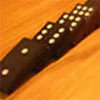### An Introduction to Mathematical Induction

##### Age 16 to 18

This article gives an introduction to mathematical induction, a powerful method of mathematical proof.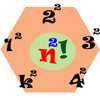### Seriesly

##### Age 16 to 18 Challenge Level:

Prove that k.k! = (k+1)! - k! and sum the series 1.1! + 2.2! + 3.3! +...+n.n!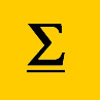### The Kth Sum of N Numbers

##### Age 16 to 18

Yatir from Israel describes his method for summing a series of triangle numbers.### 2^n -n Numbers

##### Age 16 to 18

Yatir from Israel wrote this article on numbers that can be written as $2^n-n$ where n is a positive integer.### Googol

##### Age 16 to 18 Short Challenge Level:

Find the smallest value for which a particular sequence is greater than a googol.### OK! Now Prove It

##### Age 16 to 18 Challenge Level:

Make a conjecture about the sum of the squares of the odd positive integers. Can you prove it?### Summats Clear

##### Age 16 to 18 Challenge Level:

Find the sum, f(n), of the first n terms of the sequence: 0, 1, 1, 2, 2, 3, 3........p, p, p +1, p + 1,..... Prove that f(a + b) - f(a - b) = ab.### Seriesly

##### Age 16 to 18 Short Challenge Level:

Prove that k.k! = (k+1)! - k! and sum the series 1.1! + 2.2! + 3.3! +...+n.n!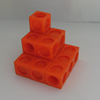### Sums of Powers - A Festive Story

##### Age 14 to 18

A story for students about adding powers of integers - with a festive twist.### The Root of the Problem

##### Age 14 to 18 Challenge Level:

Find the sum of this series of surds.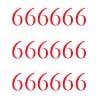### Clickety Click and All the Sixes

##### Age 16 to 18 Challenge Level:

What is the sum of: 6 + 66 + 666 + 6666 ............+ 666666666...6 where there are n sixes in the last term?### Sum the Series

##### Age 16 to 18

This article by Alex Goodwin, age 18 of Madras College, St Andrews describes how to find the sum of 1 + 22 + 333 + 4444 + ... to n terms.### Summing Geometric Progressions

##### Age 14 to 18 Challenge Level:

Watch the video to see how to sum the sequence. Can you adapt the method to sum other sequences?### Speedy Summations

##### Age 16 to 18 Challenge Level:

Watch the video to see how to add together an arithmetic sequence of numbers efficiently.### A Swiss Sum

##### Age 16 to 18 Challenge Level:

Can you use the given image to say something about the sum of an infinite series?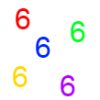### Clickety Click

##### Age 16 to 18 Short Challenge Level:

What is the sum of: 6 + 66 + 666 + 6666 ............+ 666666666...6 where there are n sixes in the last term?### Vanishing Point

##### Age 14 to 18 Challenge Level:

How can visual patterns be used to prove sums of series?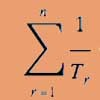### Reciprocal Triangles

##### Age 16 to 18 Challenge Level:

Prove that the sum of the reciprocals of the first n triangular numbers gets closer and closer to 2 as n grows.### Proof Sorter - Geometric Series

##### Age 16 to 18 Challenge Level:

Can you correctly order the steps in the proof of the formula for the sum of a geometric series?### More Polynomial Equations

##### Age 16 to 18 Challenge Level:

Find relationships between the polynomials a, b and c which are polynomials in n giving the sums of the first n natural numbers, squares and cubes respectively.### Double Trouble

##### Age 14 to 16 Challenge Level:### Harmonically

##### Age 16 to 18 Challenge Level:

Is it true that a large integer m can be taken such that: 1 + 1/2 + 1/3 + ... +1/m > 100 ?### Production Equation

##### Age 16 to 18 Challenge Level:

Each week a company produces X units and sells p per cent of its stock. How should the company plan its warehouse space?### Slick Summing

##### Age 14 to 16 Challenge Level:

Watch the video to see how Charlie works out the sum. Can you adapt his method?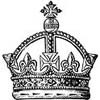### Succession in Randomia

##### Age 16 to 18 Challenge Level:

By tossing a coin one of three princes is chosen to be the next King of Randomia. Does each prince have an equal chance of taking the throne?### Degree Ceremony

##### Age 16 to 18 Challenge Level:

What does Pythagoras' Theorem tell you about these angles: 90°, (45+x)° and (45-x)° in a triangle?### Overarch 2

##### Age 16 to 18 Challenge Level:

Bricks are 20cm long and 10cm high. How high could an arch be built without mortar on a flat horizontal surface, to overhang by 1 metre? How big an overhang is it possible to make like this?### Picture Story

##### Age 14 to 16 Challenge Level:

Can you see how this picture illustrates the formula for the sum of the first six cube numbers?### Summing Squares

##### Age 14 to 16 Challenge Level:

Discover a way to sum square numbers by building cuboids from small cubes. Can you picture how the sequence will grow?